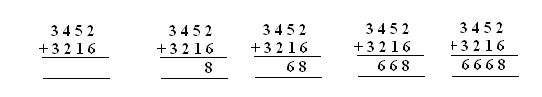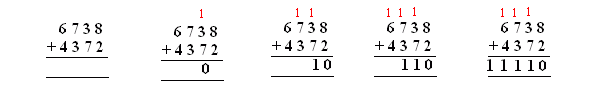Email us to get an instant 20% discount on highly effective K-12 Math & English kwizNET Programs!

#### Online Quiz (WorksheetABCD)

Questions Per Quiz = 2 4 6 8 10

 Method: Add the numbers in ones place. Move on the tens, hundreds and thousands. Example: Addition without carryStart from ones place, 6 and 2 gives 8 In tens place 5 and 1 add up to 6. In hundreds place 4 and 2 give 6. In thousands place 3 and 3 give 6. Answer: 6668 Addition with carryIn ones place, sum is 10, 1 is carried to tens place. In tens place sum is 10, add the carry. This results in 11 with a carry 1 to hundreds place. In hundreds place sum is 10 , add the carry. This results in 11 with a carry 1 to thousands place. In ten thousands place, sum is 10 , add the carry, the result is 11. Answer: 11116 Directions: Add the following four digit numbers. Also write at least ten examples for additon without carry and addition with carry.
 Question 1: This question is available to subscribers only! Question 2: This question is available to subscribers only!